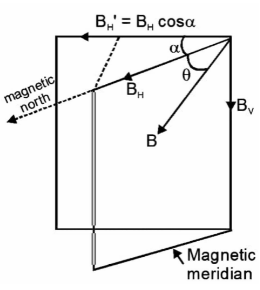Courses
Courses for Kids
Free study material
Offline Centres
MoreLast updated date: 02nd Dec 2023
Total views: 281.4k
Views today: 5.81k

# A dip needle lies initially in the magnetic meridian when it shows an angle of dip $\theta$ at a place. The dip circle is rotated through an angle $x$ in the horizontal plane and then it shows an angle of dip ${\theta ^1}$. Then$\dfrac{{\tan {\theta ^1}}}{{\tan \theta }}$ is ?Verified
281.4k+ views
Hint: Let us get some idea about meridian. A (geographic) meridian (or line of longitude) is half of an imaginary great circle on the Earth's surface, a coordinate line terminating at the North Pole and the South Pole, linking points of equal longitude measured in angular degrees east or west of the Prime Meridian.

Now let us understand about the magnetic meridian. The magnetic meridian is the vertical plane that passes across the magnetic axis of a freely suspended small magnet. The magnetic field of the earth is directed in the direction of the magnetic meridian.

Let us come to this diagram and understand the answer of the problem.In first case
$\tan \theta = \dfrac{{{B_v}}}{{{B_H}}}........(1)$
In second case
$\tan {\theta ^1} = \dfrac{{{B_v}}}{{{B_H}\cos x}}....(2)$
Form (1) and (2) we get
$\therefore \dfrac{{\tan {\theta ^1}}}{{\tan \theta }} = \dfrac{1}{{\cos x}}$

Hence, the value of $\dfrac{{\tan {\theta ^1}}}{{\tan \theta }}$ is $\dfrac{1}{{\cos x}}$.

Note:So in short we can say that the magnetic axis is a straight line that connects the Earth's magnetic poles. The magnetic meridian is a vertical plane that passes around the magnetic axis, and the magnetic equator is a large circle that is perpendicular to the Earth's magnetic axis.